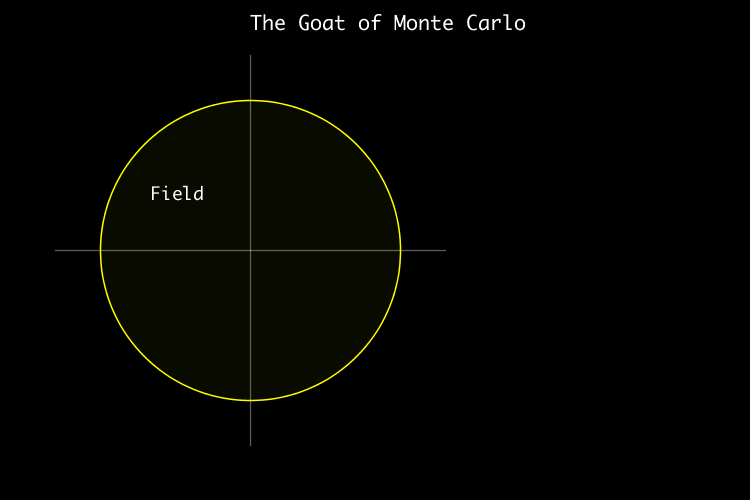# The Goat of Monte Carlo

## 24 Dec 2020

Although it has been several years since I wrote my last personal blog post, the prospect of writing about a centuries old mathematical problem involving goats, and my solution to it in Clojure, was too tempting to resist!

## The Grazing Goat Problem

The Grazing Goat Problem is a recreational mathematical problem, a form of which was first described over 270 years ago in the 1748 issue of the interestingly titled publication The Ladies Diary: Or, The Woman’s Almanack.

The problem is described as follows:

Imagine a circular fence that encloses an area of grass. If you tie a goat to the inside of the fence, how long a rope do you need to allow the animal access to exactly half that area?

At first blush it seems like a simple problem to solve, and yet an exact solution eluded mathematicians for centuries. Given the length of rope, working out the area of grass the goat has access to is straightforward and well described as a circle-circle intersection problem. However working out the reverse i.e. the length of rope required for the goat to access a given area, wasn’t so easy… that is, until an exact solution was found this year.

The fact that the problem revolves around calculating the ratio of two areas led me to explore an alternative solution.

## The Monte Carlo Method

The Monte Carlo Method, named after the famous casino in Monte Carlo, involves using random sampling to arrive at an approximate solution to a problem. The most commonly described example using this method involves the calculation of π, where the quadrant of a circle is inscribed within a square, then dots are randomly scattered within the square.Monte Carlo Method applied to approximating the value of π. Image courtesy of nicoguaro (Wikipedia) CC BY 3.0.

The ratio of the number of dots landing inside the quadrant as a proportion of the total number of dots approximates π/4. The greater the number of random dots fired, the more likely the result will tend toward the exact solution.

To apply this technique to the Grazing Goat Problem, a circular field is inscribed within a square, then dots are randomly scattered within the square for multiple trials of rope length. For each trial, calculate the ratio of the number of dots landing in the roped area as a proportion of the number of dots landing in the full fenced area. When that proportion is 0.5, we have our solution.Grazing Goat Problem solved using the Monte Carlo Method

I wrote a quick Clojure solution to the problem where the resulting length of the rope is expressed as a multiple of the radius of the enclosed area. The number of random dots fired is 10,000 to balance speed with accuracy. The ratio found is around 1.155 compared to the “exact” answer of 1.15872847.

## A Little Bit of Code

Here’s a quick rundown of the main parts of the code.

1. Try rope lengths from 1.0 to 1.25 in 0.01 increments:
(defn run []
(for [rope-len (range 1.0 1.25 0.01)]
(let [ratio (calculate-ratio (generate-outcomes 10000 rope-len))]
{:rope-len rope-len :ratio ratio})))

1. Generate a bunch of random points recording whether each point lands inside the field or just the roped area:
(defn generate-outcomes [trials rope-len]
(map
(fn [point] {:rope (point-in-rope? rope-len point) :field (point-in-field? point)})
(random-points trials)))

1. Testing whether a point lands inside the field or the roped area uses Pythagoras' Theorem:
(defn point-in-circle? [centre-x centre-y r [point-x point-y]]
(let [x (- point-x centre-x)
y (- point-y centre-y)]
(< (+ (square x) (square y)) (square r))))

1. And the final result is the ratio of points inside the roped area to points inside the whole field:
(defn calculate-ratio [outcomes]
(let [in-field (filter :field outcomes)
in-rope (filter :rope in-field)]
(/ (count in-rope) (count in-field))))


The full source can be found here.